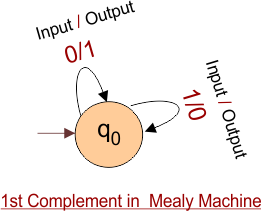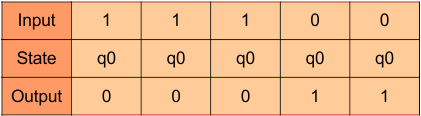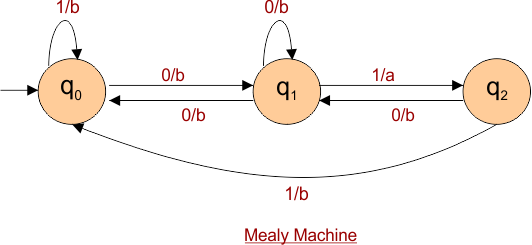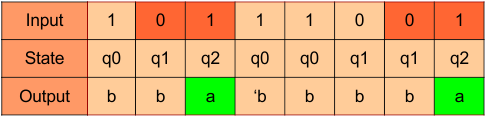Select Page

# Examples of Automata Mealy Machine

As we already covered the topic of Automata Mealy Machine. In this session we will see some examples of Automata Mealy Machine.

## Example 1

Design a Moore machine to generate 1’s complement of a any given binary input.

### Solution

According to first complement, if the given input is “1” then the output will be “0” and vice versa.

Hence, the Mealy Machine of given question is given belowFor instance, take one binary number 11100 then look at the following tableThus we get 00011 which is 1’s complement of 11100,

## Example 2

Design a Mealy Machine that prints “a” whenever the sequence “01” is encountered in any input binary string.

### Solution

The requirement is that, whenever the “01” appear in the string the output must be end with “a”

Desired Mealy Machine is given belowFor instance, take one binary number 10111001 then look at the following tableAs shown in the above table, whenever the “01” occurs in input string then the output will be “a”

Help Other’s By Sharing…